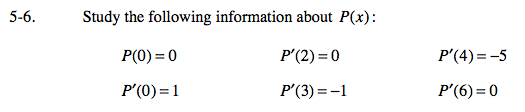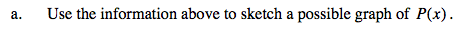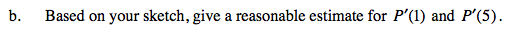### Home > CALC > Chapter 5 > Lesson 5.1.1 > Problem5-6

5-6.
1. Study the following information about P(x): Homework Help ✎

 P(0) = 0 P ′(2) = 0 P ′(4) = −5 P ′(0) = 1 P ′(3) = −1 P ′(6) = 0

1. Use the information above to sketch a possible graph of P(x).

2. Based on your sketch, give a reasonable estimate for P ′(1) and P ′(5).Plot points in reasonable areas and draw short lines to represent the slope at each point.Use your graph to guess what the slope is at P(1) and P(5).

Use the eTool below to help solve the problem.
Click the link at right for the full version of the eTool: Calc 5-6 HW eTool